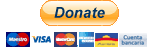# skforecast¶

Time series forecasting with scikit-learn regressors.

Skforecast is a python library that eases using scikit-learn regressors as multi-step forecasters. It also works with any regressor compatible with the scikit-learn API (pipelines, CatBoost, LightGBM, XGBoost, Ranger...).

## Installation¶

The default installation of skforecast only installs hard dependencies.

 `1` ``````pip install skforecast ``````

Specific version:

 `1` ``````pip install skforecast==0.6.0 ``````

Latest (unstable):

 `1` ``````pip install git+https://github.com/JoaquinAmatRodrigo/skforecast#master ``````

Install the full version (all dependencies):

 `1` ``````pip install skforecast[full] ``````

Install optional dependencies:

 `1` ``````pip install skforecast[statsmodels] ``````
 `1` ``````pip install skforecast[plotting] ``````

## Dependencies¶

### Hard dependencies¶

• numpy>=1.20, <1.24
• pandas>=1.2, <1.6
• tqdm>=4.57.0, <4.65
• scikit-learn>=1.0, <1.2
• optuna>=2.10.0, <3.1
• scikit-optimize==0.9.0
• joblib>=1.1.0, <1.3.0

### Optional dependencies¶

• matplotlib>=3.3, <3.7
• seaborn==0.11
• statsmodels>=0.12, <0.14

## Features¶

• Create recursive autoregressive forecasters from any regressor that follows the scikit-learn API
• Create direct autoregressive forecasters from any regressor that follows the scikit-learn API
• Create multi-time series autoregressive forecasters from any regressor that follows the scikit-learn API
• Include exogenous variables as predictors
• Include custom predictors (rolling mean, rolling variance ...)
• Multiple backtesting methods for model validation
• Grid search, random search and bayesian search to find optimal lags (predictors) and best hyperparameters
• Include custom metrics for model validation and grid search
• Prediction interval estimated by bootstrapping and quantile regression
• Get predictor importance
• Forecaster in production

## Donating¶

If you found skforecast useful, you can support us with a donation. Your contribution will help to continue developing and improving this project. Many thanks!